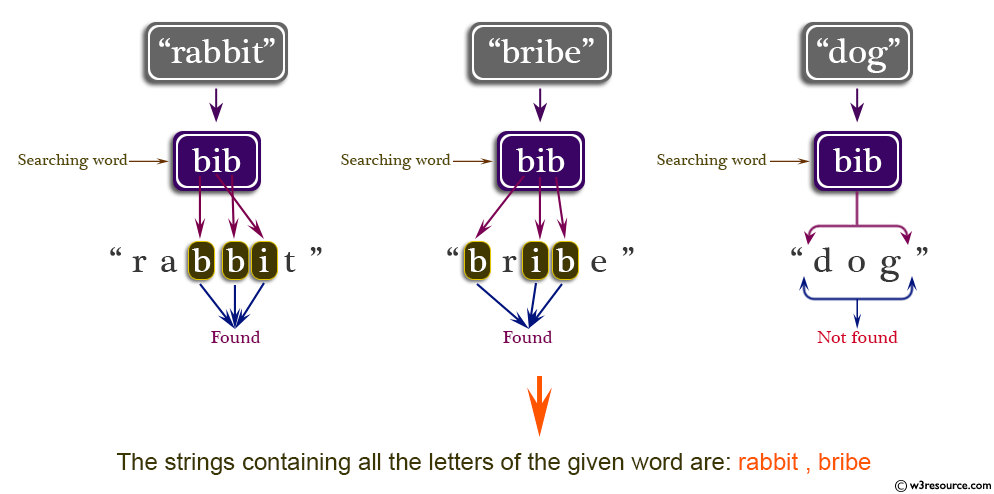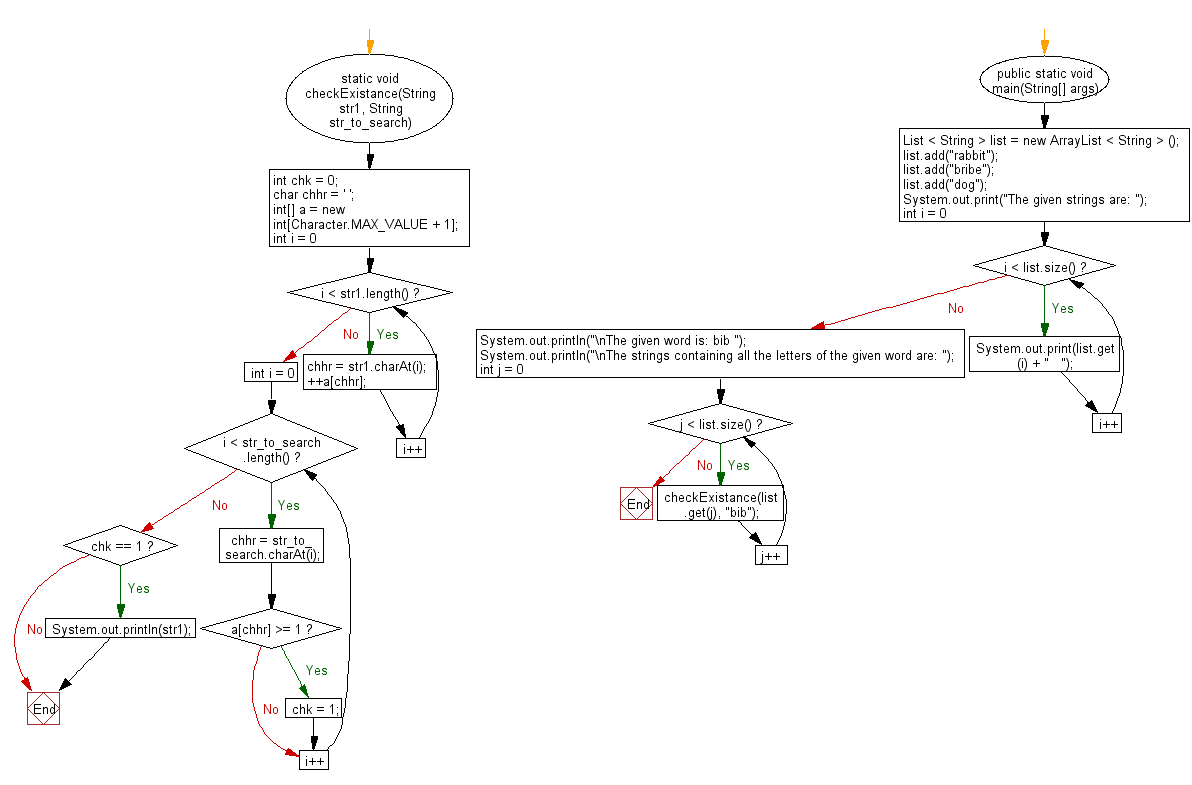﻿ Java exercises: Print list items containing all characters of a specified word - w3resource# Java String Exercises: Print list items containing all characters of a specified word

## Java String: Exercise-42 with Solution

Write a Java program to print list items containing all characters of a given word.

Pictorial Presentation:Sample Solution:

Java Code:

``````import java.util.*;
class Main {
static void checkExistance(String str1, String str_to_search) {
int chk = 0;
char chhr = ' ';
int[] a = new int[Character.MAX_VALUE + 1];

for (int i = 0; i < str1.length(); i++) {
chhr = str1.charAt(i);
++a[chhr];
}
for (int i = 0; i < str_to_search.length(); i++) {
chhr = str_to_search.charAt(i);
if (a[chhr] >= 1)
chk = 1;
}
if (chk == 1)
System.out.println(str1);
}

public static void main(String[] args) {
List < String > list = new ArrayList < String > ();
list.add("rabbit");
list.add("bribe");
list.add("dog");
System.out.print("The given strings are: ");
for (int i = 0; i < list.size(); i++) {
System.out.print(list.get(i) + "   ");
}
System.out.println("\nThe given word is: bib ");
System.out.println("\nThe strings containing all the letters of the given word are: ");
for (int j = 0; j < list.size(); j++) {
checkExistance(list.get(j), "bib");
}
}
}
```
```

Sample Output:

```The given strings are: rabbit   bribe   dog
The given word is: bib

The strings containing all the letters of the given word are:
rabbit
bribe
```

Flowchart:Java Code Editor:

Improve this sample solution and post your code through Disqus

What is the difficulty level of this exercise?

﻿